# How To Generate Random Number in Python

Python random module provides access to functions that support many operations that generate random numbers. It is beneficial when we want the computer to pick a random number in a given range. Python random module implements pseudo-random number generators for various distributions.

For integers, it can generate a uniform selection from a range. For sequences, it can create a uniform selection of a random element.

For a function, it can generate a random permutation of a list in-place and a function for random sampling without replacement.

## How To Generate Random Number in Python

Python random module allows you to create random values and generate random choices.

Let’s take a simple example to generate a random value between 0 to 1.

```# app.py

import random

print('The random value between 0 to 1 is:', random.random())```

See the output.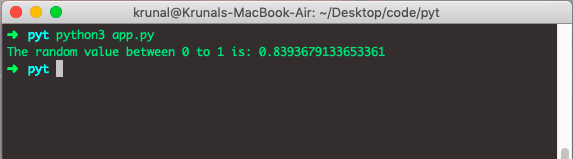## Python random.randrange Function

Now, let’s see an example of a randrange function. See the below code.

We will print the number between 1 to 10.

```# app.py

import random

print('The random value between 1 to 10 is:', random.randrange(1,10))```

Each time, we execute the above code, we will get a different output.

See the output below.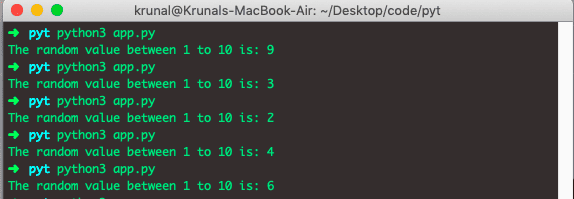In the above example, we have taken the range from 1 to 10. So, here the 10 is excluded. It will only print from 1 to 9.

Please keep in mind that the upper max will not print in the output. With random.randrange() function, you can exclude the right-hand side of the interval, meaning the generated number always lies within [x, y) and will always be smaller than the right endpoint.

## Generate Random items in List

Let’s write the following code where we can output the random items on the list.

```# app.py

import random

print('The random items of list is:',random.sample(range(22), 6))```

See the output.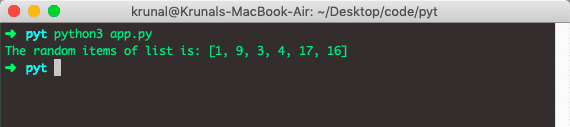## Generate Random Choices in Python

If we want to pick a random item from the non-empty sequence like a list or a tuple, you can use random.choice().

There is also random.choices() function for choosing multiple elements from a sequence with replacement (duplicates are possible). Let’s take a list of items and then, write a choice function to give a choice from those items.

```# app.py

import random

Disney = ['Moana', 'Alladin', 'Lion King', 'Tangled', 'Pinnochio', 'Cars']
print('Let\'s see this movie called', random.choice(Disney))```

See the following output.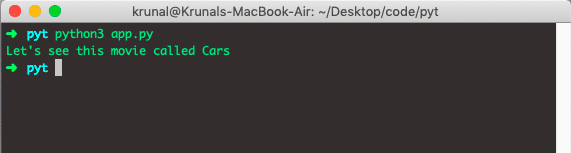## Python random.shuffle Example

You can randomize a sequence in-place using random.shuffle() function. This will modify the sequence object and randomize the order of elements. The random.shuffle() function helps us to shuffle the objects in the list, tuple, or dictionary. See the below example.

```# app.py

import random

Pixar = ['Up', 'Toy Story', 'Coco', 'Inside out', 'Brave', 'Finding Dory']
random.shuffle(Pixar)
print(Pixar)```

See the output.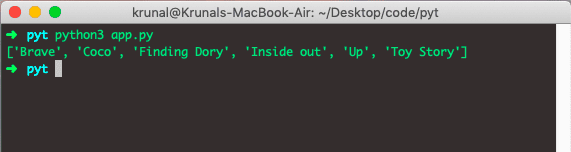You can keep shuffling them, and it will display the different variations.

## Python random.randint Example

Let’s see an example of Python random.randint function example.

It is the same as random.randrange function but, it will include both endpoints as well.

```# app.py

import random

print(random.randint(1, 10))```

See the below.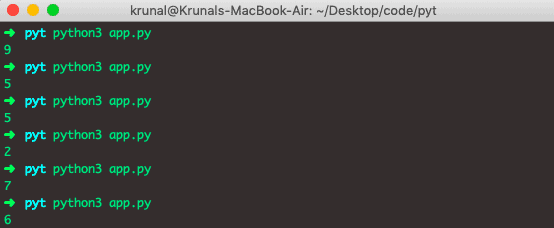## Python random.uniform Example

If you need to generate the random floats that lie within the specific [x, y] interval, you can use the random.uniform(), which plucks from the continuous uniform distribution.

See the following code to generate a number.

```# app.py

import random

print(random.uniform(1, 5))```

See the output.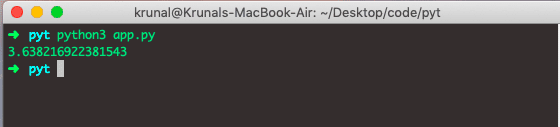## All in one table

Package/Module Description Cryptographically Secure
random Fasty and easy random data No
numpy.random Like random but for possibly multidimensional arrays No
os Contains urandom(), a base of other functions covered here Yes
secrets Designed to be Python’s de-facto module for generating secure random numbers, bytes, and strings Yes
UUID It is a module with a handful of functions for building 128-bit identifiers Yes, uuid4()

Finally, How To Generate Random Number in Python Tutorial With Example is over.

This site uses Akismet to reduce spam. Learn how your comment data is processed.